# Wire Grilles & Panels

Van Dyke’s wire grille cabinet panel inserts from brands like Kent Designs, add gleaming metallic beauty to cabinets, hutches, entertainment centers, and more. Choose from dozens of styles. Another popular choice is our textured glass in choice of colors and patterns, You will also find opaque choices like aged solid copper sheets and wooden veneer in finishes like Walnut, African Mahogany, Oak, and Birdseye Maple.

•As low as:
\$257.63 Each

•SALE as low as:
\$82.61 Each

•SALE as low as:
\$41.04 Each

•As low as:
\$192.09 Each

•As low as:
\$0.41 Per Square Inch

•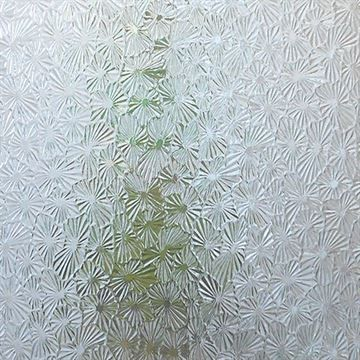As low as:
\$0.33 Per Square Inch

•As low as:
\$0.41 Per Square Inch

•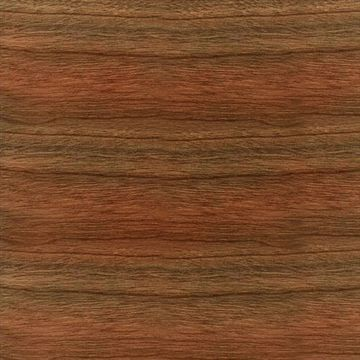SALE as low as:
\$61.68 Each

•As low as:
\$196.61 Each

•As low as:
\$149.15 Each

•As low as:
\$0.33 Per Square Inch

•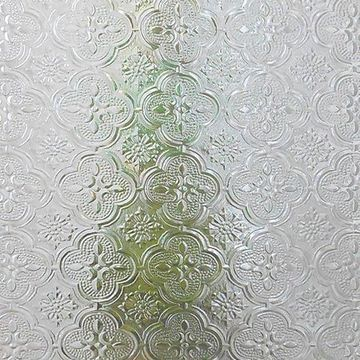As low as:
\$0.29 Per Square Inch

•SALE as low as:
\$57.82 Each

•As low as:
\$0.29 Per Square Inch

•As low as:
\$0.41 Per Square Inch

•As low as:
\$0.33 per Square Inch

•SALE as low as:
\$8.87 Each

•SALE as low as:
\$9.64 Each

•SALE as low as:
\$98.66 Each

•As low as:
\$0.29 Per Square Inch

•As low as:
\$0.29 Per Square Inch

•As low as:
\$0.33 Per Square Inch

•SALE as low as:
\$6.17 Each

•As low as:
\$0.29 Per Square Inch

•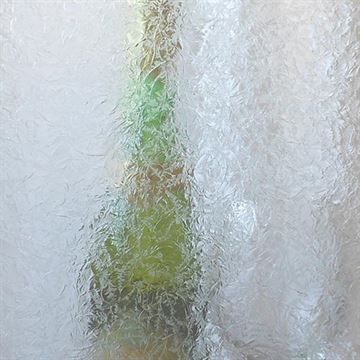As low as:
\$0.33 Per Square Inch

•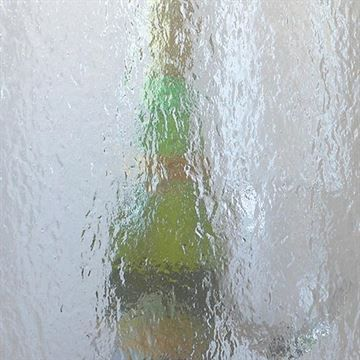As low as:
\$0.33 Per Square Inch

•SALE as low as:
\$55.37 Each

•As low as:
\$0.29 Per Square Inch

•SALE as low as:
\$60.38 Each

•As low as:
\$9.19 Each

•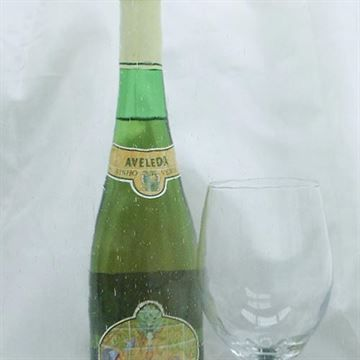As low as:
\$0.41 Per Square Inch

•As low as:
\$0.33 Per Square Inch

•SALE as low as:
\$6.95 Each

•SALE as low as:
\$37.20 Each

•SALE as low as:
\$6.17 Each

•As low as:
\$0.33 Per Square Inch

•As low as:
\$201.13 Each

•As low as:
\$0.33 Per Square Inch

•SALE as low as:
\$6.95 Each

•As low as:
\$0.41 Per Square Inch

•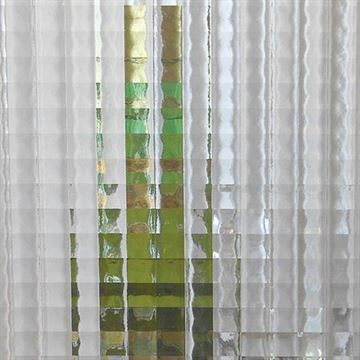As low as:
\$0.29 Per Square Inch

•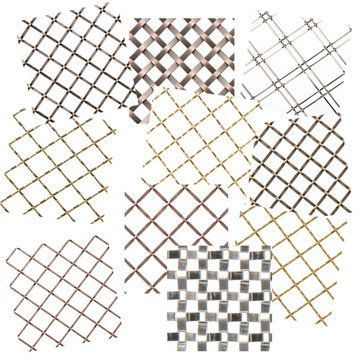As low as:
\$0.99 Each

•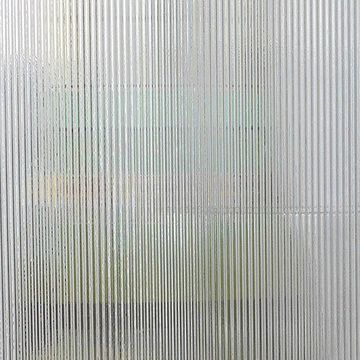As low as:
\$0.29 Per Square Inch

•As low as:
\$0.33 Per Square Inch

•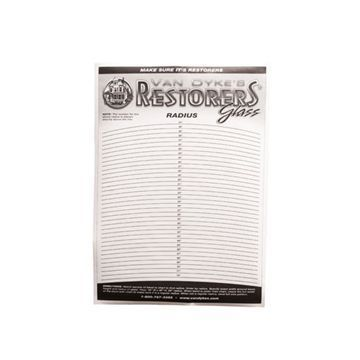As low as:
\$8.99 Each

•As low as:
\$0.29 Per Square Inch

•As low as:
\$0.33 Per Square Inch

•As low as:
\$9.19 Each